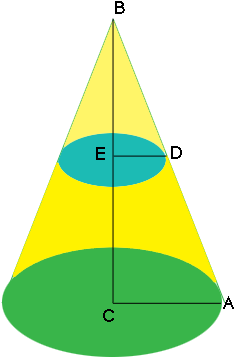SEARCH HOMEMath Central Quandaries & QueriesQuestion from c.j, a student: what is the length of the radius of the LARGER cone(the LARGER cone has a slant height of 15) when the SMALLER cone has a radius of 8 and a slant height of 12ft ,please help.Hi,

I assume have a diagram that looks something like this.If so then the triangles $ABC$ and $DBE$ are similar and thus

$\frac{|AB|}{|BC|} = \frac{|DB|}{|BE|}$

I hope this helps,
PennyMath Central is supported by the University of Regina and The Pacific Institute for the Mathematical Sciences.